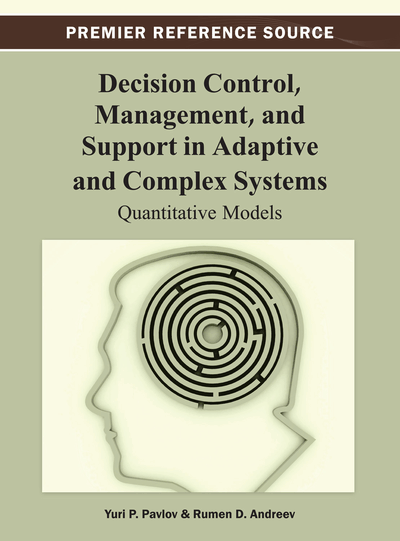# Elements of Utility Theory

DOI: 10.4018/978-1-4666-2967-7.ch004

## Abstract

In the science literature there are many examples of investigation and application of the theory of measurements and utility theory in different areas of human activity. The authors use in the following chapters their achievements for applications in optimal control of bioprocesses for a complete mathematical description of complex systems “technologist- dynamical process,” and in the agriculture for modeling difficult examples and for formalizing another way.
Chapter Preview
Top

## 1. Beginnings: The St. Petersburg Paradox

In the previous chapter, we presented the fundamental concepts in the theory of measurement and scaling. There we defined the term value or utility, as a measurement scale of the preferences of the decision maker. Let (X, (R)) is a system with relations (SR) defined over the set X together with preference relation over it (xy) (R≡(), (strict preference or strict order or strong order, weak order and weak connectedness). In this manner, a structure is introduced in the set X, (X, ()). If there exists homomorphism u(.):(X, ())→(R, (>)), then this homomorphism will be called value function. In the above formulas, we denote by R the set of the real numbers or a subset of it, and with (>) the relation greater, defined over the real numbers. Following (Pfanzagl, 1971) this homomorphism is defined as follows:

((x, y)∈X2, xy) ⇔ (u(x)>u(y)).

This value function is also called perfect value function. If the homomorphism is defined by the inclusion (X⊆u-1(R)), then the definition of value function is the following:

((x, y)∈X2, xy) ⇒ (u(x)>u(y)).

In other words, the value function is a homomorphism from the empiric system (Decision Maker (DM), preferences) to (subset of the set of the real numbers with relation greater). We assume that the set X is countable, i.e. bijective to the set of the natural numbers (the set of integers). Then the following propositions are fulfilled (Fishburn, 1970).

### 1.1. Proposition

Value function u(.) for the preference relation () of X exists if and only if the relation is acyclic (i.e. there does not exist (x1, x2, x3, ....,xh), for which (x1x2, x2x3, x3x4, ....,xh-1xh, xhx1)).

This proposition is obvious and follows directly from the properties of the relation (>) defined over the real numbers and the definition of the homomorphism. We define the relation “indifference, indiscernibility or equivalence” as ((x≈y) ⇔ ¬((xy) ∨ (xy))). In Fishburn (1970), the following theorem is proved.

### 1.2. Theorem

Let the relation () is weak order (asymmetric and negatively transitive relation) over the set of alternatives. Then it is fulfilled:

For any two alternatives (x, y) one of the following relations is fulfilled (xy), (yx) or (x≈y):

• The relation () is transitive;

• The relation () is equivalence;

• ((xy)∧ (y≈z)) ⇒ (xz), ((x≈y)∧ (yz)) ⇒ (xz);

• The relation ((xy)∨(x≈y)) “more preferred or equivalent” is transitive and connected;

The relation (’) over the factor set (X/) is strong order (weak connected weak order) (a, bX/≈, (a’b) ⇔ (∃ x, yX, such that (xa)∧ (yb) ∧(xy)).

We investigated part of the above propositions in the previous chapter 3. We saw that asymmetricity and the negative transitivity lead to the transitivity of the preference relation () and transitivity of (). Now we will state a fundamental theorem ensuring the existence of perfect value function—Fishburn’s terminology (Fishburn, 1970).

## Complete Chapter List

Search this Book:
Reset